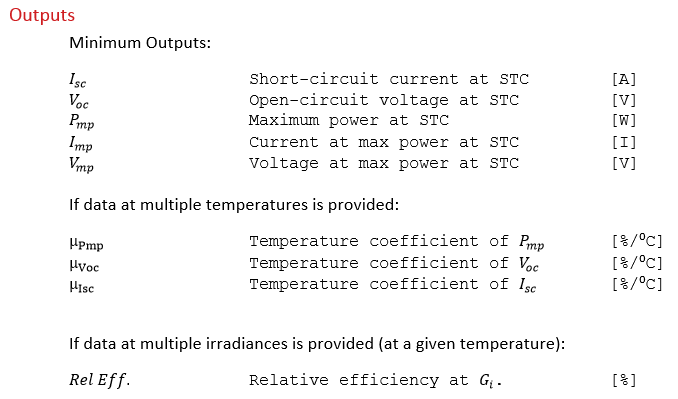# Processing Full I-V Curves

The “Full I-V Curves” input option provides another alternative “basic datasheet” parameters as input for the module file generating algorithm. This is a pre-processing step to the core module file generator.*Important Note: I-V curves intended to be provided for multiple temperature/irradiance conditions (, ). The minimum required input is data at STC.Full IV Curves Algorithm

For each I-V curve (at a unique set of temperature and irradiance conditions):

1. Short-circuit current is extracted by taking the value of current where voltage is equal to zero. If the I-V curve does not include a point at the I-intecept, the closest point is taken and an interpolation is made to the I-axis using the slope of the I-V curve nearest to the I-axis.

1. Open-circuit voltage is extracted by taking the value of voltage where current is equal to zero. If the I-V curve does not include a point at the V-intecept, the closest point is taken and an interpolation is made to the V-axis using the slope of the I-V curve nearest to the V-axis.

1. The maximum power point is determined by multiplying every set of current and voltage values together, and taking the maximum of that resulting list. The current at maximum power and voltage at maximum power are those that resulted in the maximum power point.

After the parameter extraction is performed on each provided I-V curve, the resulting data is processed as described in the documentation for “Key I-V Points” to calculate temperature coefficients and relative efficiencies.